Statement Questions

Chapter 5 Class 8 Squares and Square Roots
Concept wise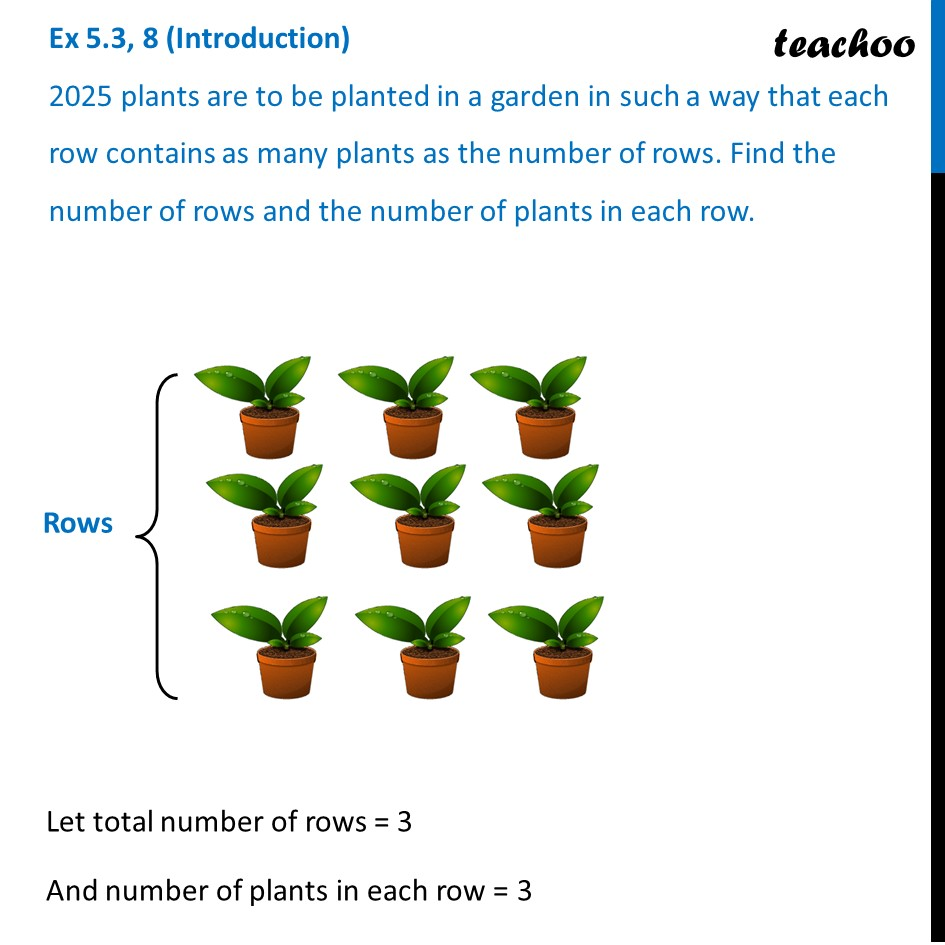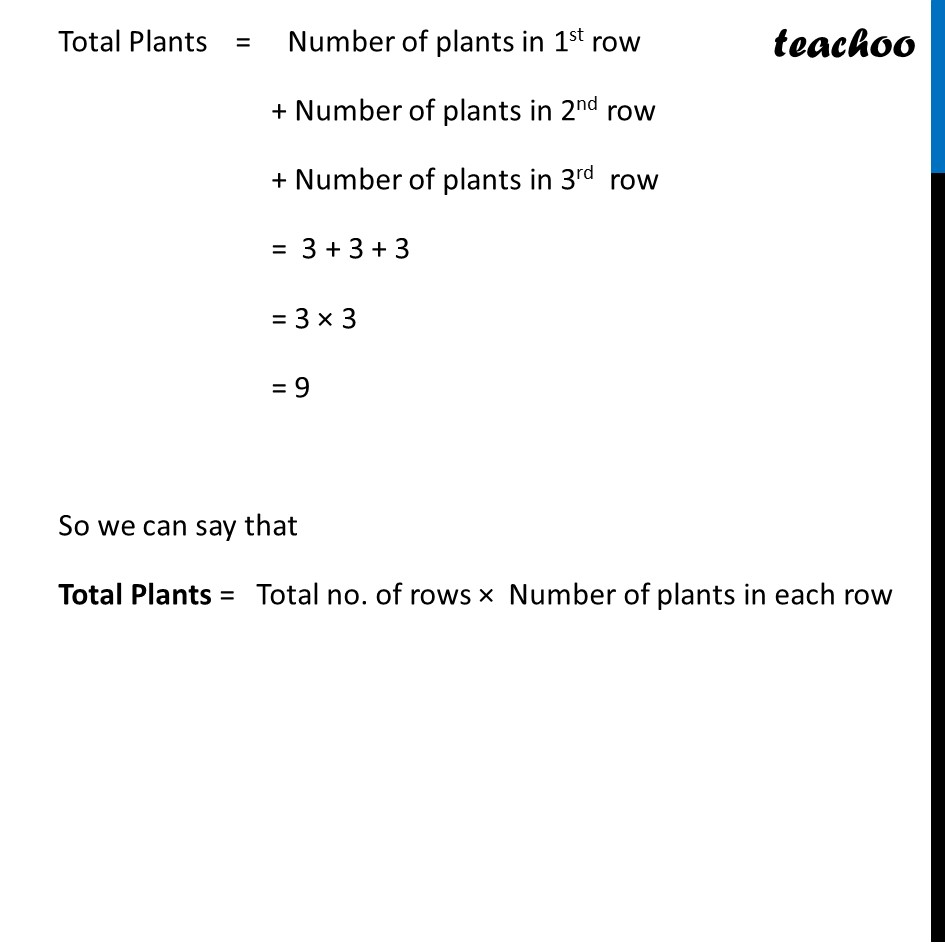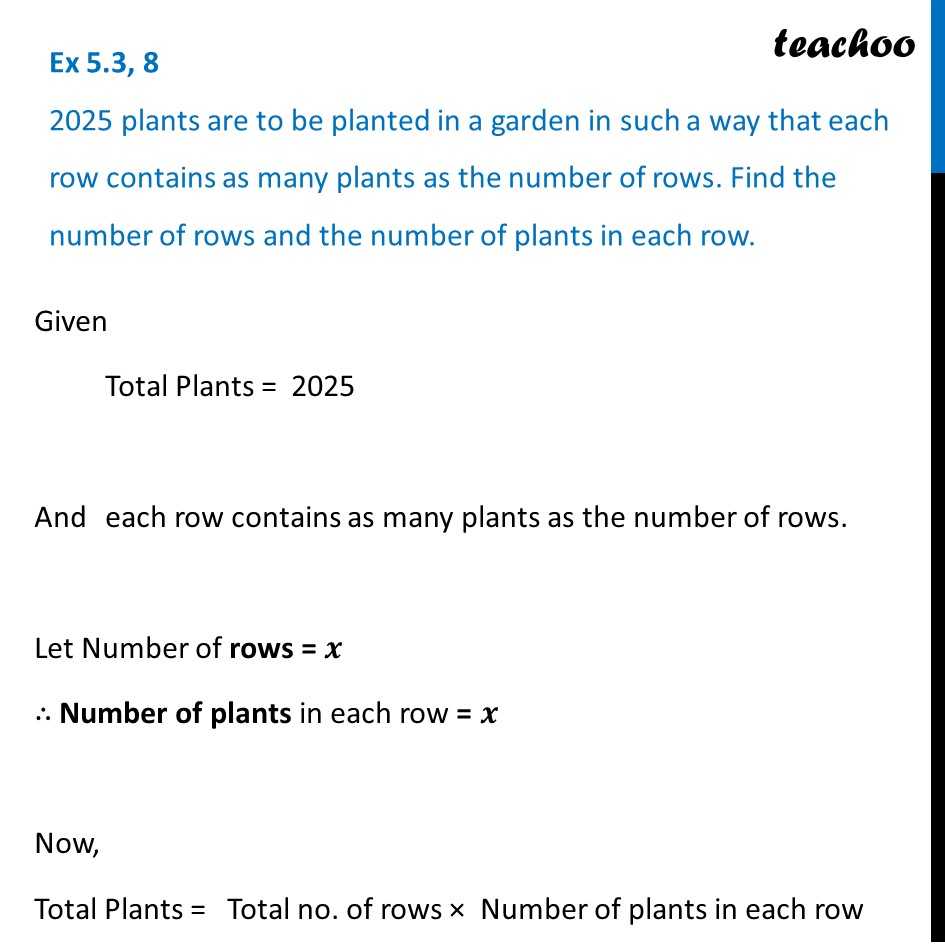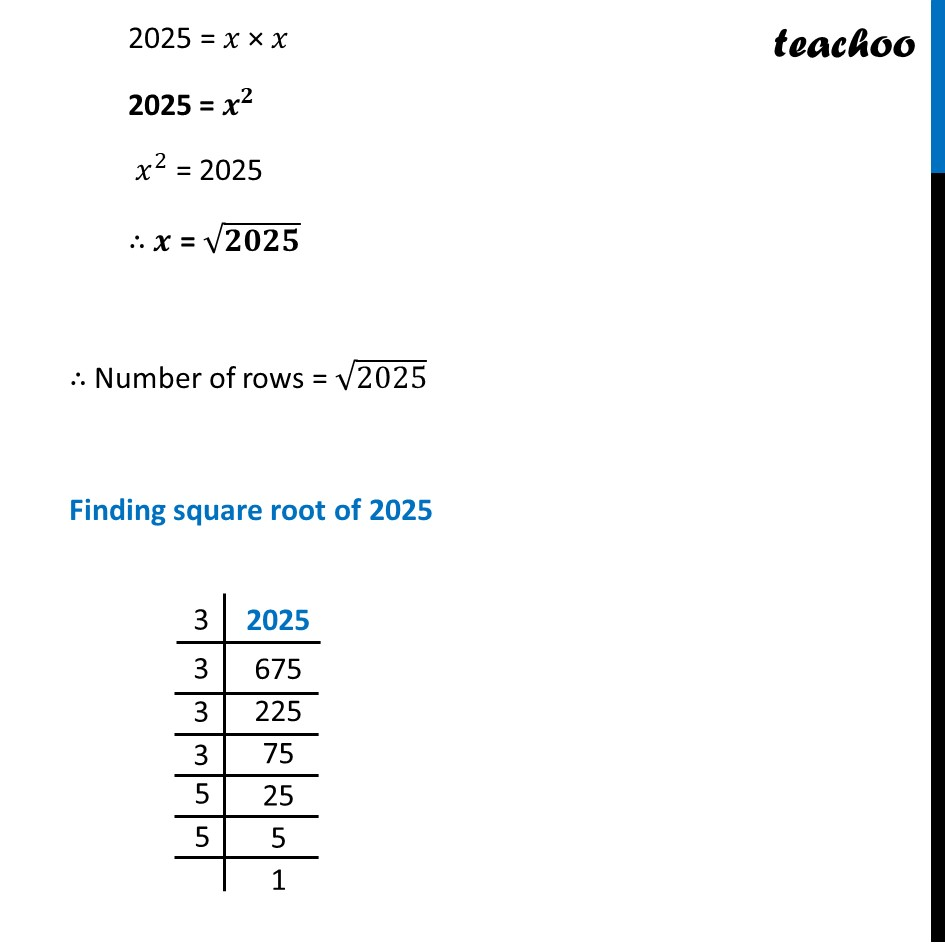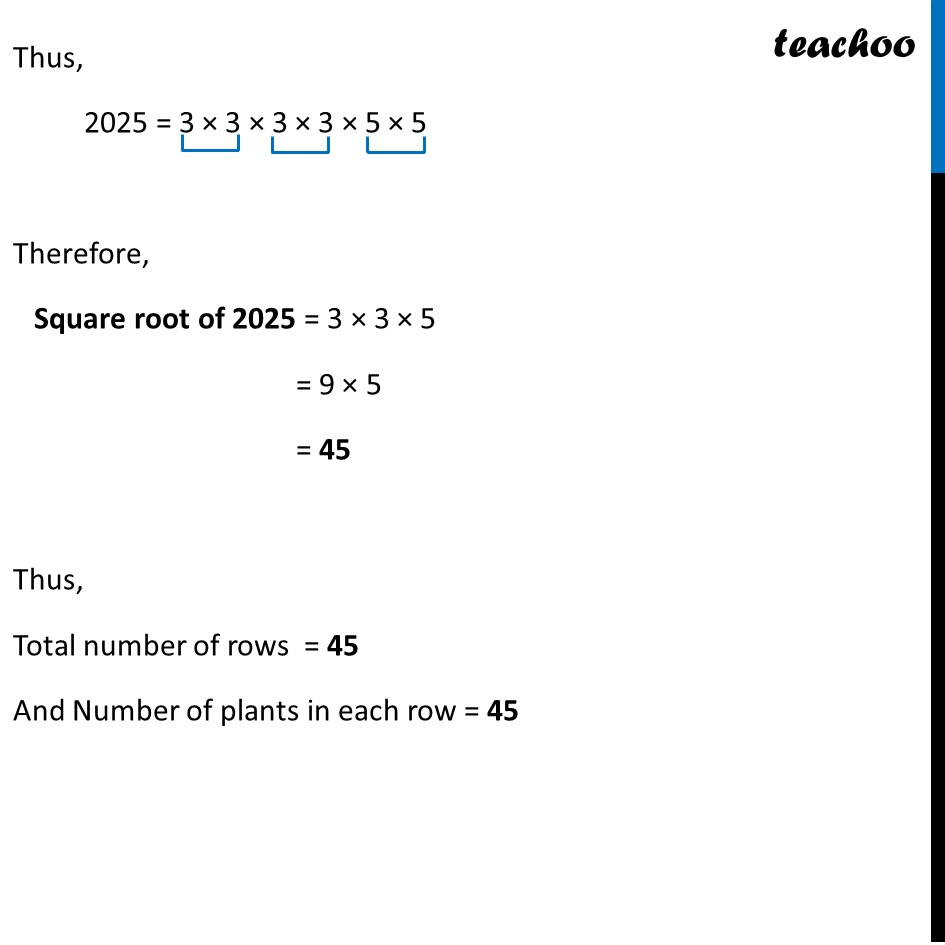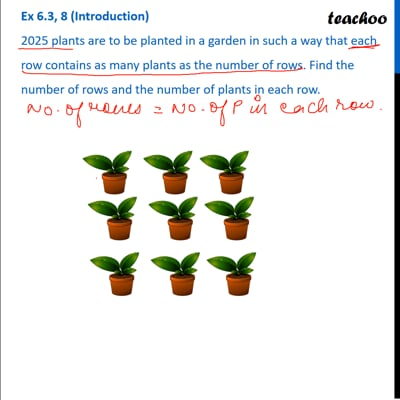This video is only available for Teachoo black users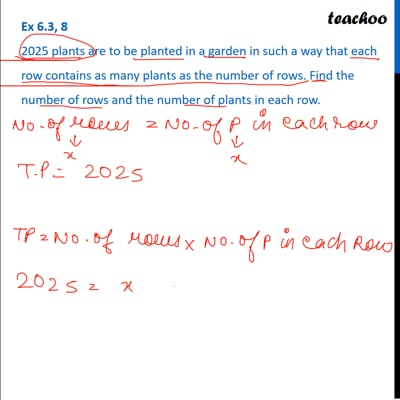This video is only available for Teachoo black users

Learn in your speed, with individual attention - Teachoo Maths 1-on-1 Class

### Transcript

Ex 5.3, 8 (Introduction) 2025 plants are to be planted in a garden in such a way that each row contains as many plants as the number of rows. Find the number of rows and the number of plants in each row. Let total number of rows = 3 And number of plants in each row = 3 Total Plants = Number of plants in 1st row + Number of plants in 2nd row + Number of plants in 3rd row = 3 + 3 + 3 = 3 × 3 = 9 So we can say that Total Plants = Total no. of rows × Number of plants in each row Ex 5.3, 8 2025 plants are to be planted in a garden in such a way that each row contains as many plants as the number of rows. Find the number of rows and the number of plants in each row. Given Total Plants = 2025 And each row contains as many plants as the number of rows. Let Number of rows = 𝒙 ∴ Number of plants in each row = 𝒙 Now, Total Plants = Total no. of rows × Number of plants in each row 2025 = 𝑥 × 𝑥 2025 = 𝒙^𝟐 𝑥^2 = 2025 ∴ 𝒙 = √𝟐𝟎𝟐𝟓 ∴ Number of rows = √2025 Finding square root of 2025 Thus, 2025 = 3 × 3 × 3 × 3 × 5 × 5 Therefore, Square root of 2025 = 3 × 3 × 5 = 9 × 5 = 45 Thus, Total number of rows = 45 And Number of plants in each row = 45